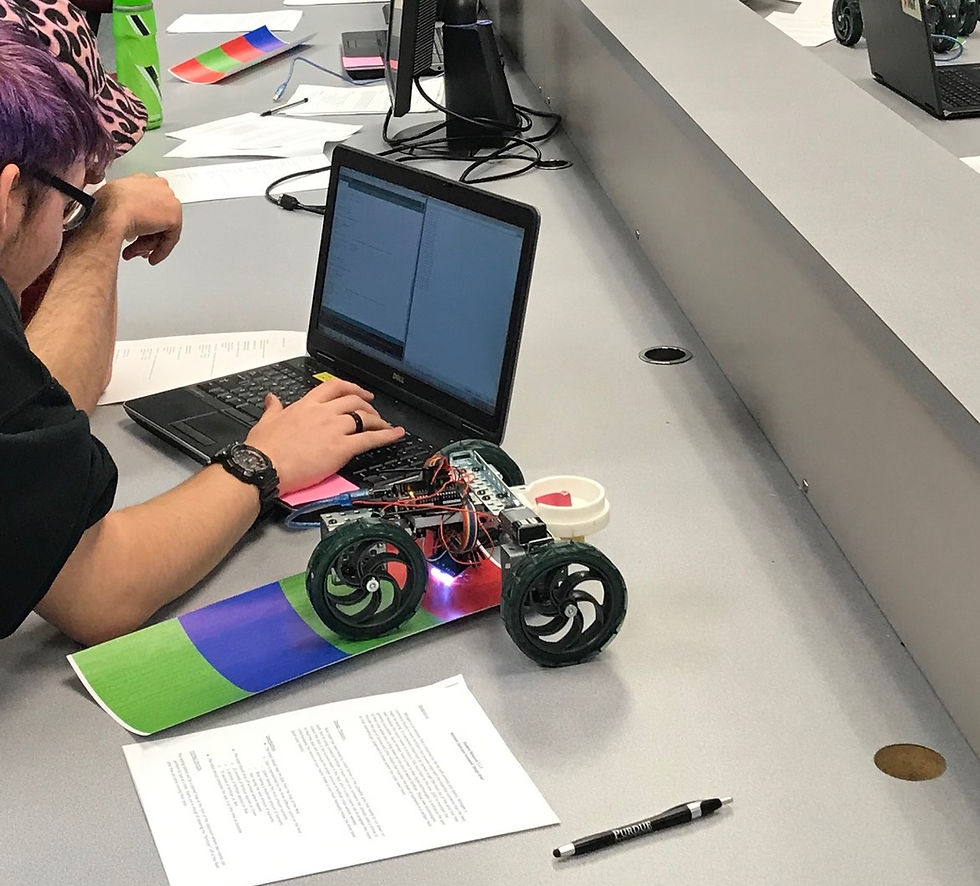top of pageEngineering Practices: Quantitative Analysis

## Computational Tools## Engineering Performance Matrix

Computational Tools are the programs, languages, and computer applications that facilitate engineering tasks which includes (a) spreadsheet tools (e.g. Microsoft Excel), (b) system design tools (e.g. LabView), and (c) computational environments (e.g. MATLAB). Computational Tools are important to the practice of Quantitative Analysis as mathematical modeling is an integral part of the engineering design process. Engineering professionals use such tools to facilitate the tasks of computing complex equations, managing large amounts of data, developing programs to process/analyze quantitative data, and communicating information. Furthermore, these tools enable users to design digital prototypes of solutions and perform statistical calculations to determine how well a solution will perform as well as why a solution performed in the way that it did.

## Performance Goal for High School Learners

I can successfully select and use the appropriate computational tools to analyze quantitative data related to an engineering problem to communicate/predict the effectiveness of a solution design.

## PROFICIENT

I can explain and demonstrate how to build simple formulas in Excel that will update automatically when data or variables are modified. (e.g. calculations using basic operations: addition, subtraction, division, multiplication, exponents, or any other simple built-in equations)

I can explain and demonstrate how to build complex formulas (in some cases, with absolute references, and can include complex calculations and nested formulas) in Excel that will update automatically when data or variables are modified.

I can explain and demonstrate how to build complex relationships in Excel that will perform one of a few types of calculations when logic is used in the Excel formula.

## SYSTEM DESIGN TOOLS(E.G. LABVIEW)

I can explain and demonstrate how to display, manipulate, and solve matrices, arrays, and variables through industry-relevant computational programs.

I can explain and demonstrate how to create functions, call functions, and create various plots of data entered or imported through industry-relevant computational programs.

I can explain and demonstrate how to create programs and scripts that will utilize various types of program components or loops (for, while, if then, if else if and/or switch case) through industry-relevant computational programs.

## COMPUTATIONAL ENVIRONMENTS(E.G. MATLAB)

I can explain and demonstrate how to display, manipulate, and solve matrices, arrays, and variables through industry-relevant computational programs.

I can explain and demonstrate how to create functions, call functions, and create various plots of data entered or imported through industry-relevant computational programs.

I can explain and demonstrate how to create programs and scripts that will utilize various types of program components or loops (for, while, if then, if else if and/or switch case) through industry-relevant computational programs.

bottom of page